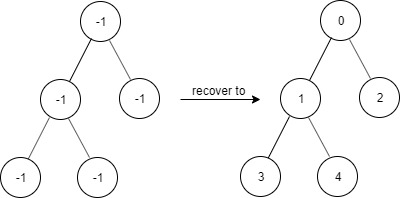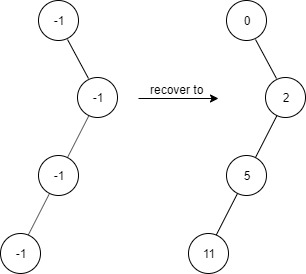Formatted question description: https://leetcode.ca/all/1261.html

# 1261. Find Elements in a Contaminated Binary Tree

Medium

## Description

Given a binary tree with the following rules:

1. root.val == 0
2. If treeNode.val == x and treeNode.left != null, then treeNode.left.val == 2 * x + 1
3. If treeNode.val == x and treeNode.right != null, then treeNode.right.val == 2 * x + 2

Now the binary tree is contaminated, which means all treeNode.val have been changed to -1.

You need to first recover the binary tree and then implement the FindElements class:

• FindElements(TreeNode* root) Initializes the object with a contamined binary tree, you need to recover it first.
• bool find(int target) Return true if the target value exists in the recovered binary tree.

Example 1:Input
["FindElements","find","find"]
[[[-1,null,-1]],,]
Output
[null,false,true]
Explanation
FindElements findElements = new FindElements([-1,null,-1]);
findElements.find(1); // return False
findElements.find(2); // return True


Example 2:Input
["FindElements","find","find","find"]
[[[-1,-1,-1,-1,-1]],,,]
Output
[null,true,true,false]
Explanation
FindElements findElements = new FindElements([-1,-1,-1,-1,-1]);
findElements.find(1); // return True
findElements.find(3); // return True
findElements.find(5); // return False


Example 3:Input
["FindElements","find","find","find","find"]
[[[-1,null,-1,-1,null,-1]],,,,]
Output
[null,true,false,false,true]
Explanation
FindElements findElements = new FindElements([-1,null,-1,-1,null,-1]);
findElements.find(2); // return True
findElements.find(3); // return False
findElements.find(4); // return False
findElements.find(5); // return True


Constraints:

• TreeNode.val == -1
• The height of the binary tree is less than or equal to 20
• The total number of nodes is between [1, 10^4]
• Total calls of find() is between [1, 10^4]
• 0 <= target <= 10^6

## Solution

In the constructor, recover the tree using breadth first search. The root’s value is 0. For each node that is not a leaf, obtain its two children, and if a child is not null, recover the child’s value.

In the method boolean find(int target), first obtain the path from the root to the target, and then check whether there exists a node with value target. The path can be obtained using the relations between the parent’s value and its children’s values. To find the node, also use the relations.

/**
* Definition for a binary tree node.
* public class TreeNode {
*     int val;
*     TreeNode left;
*     TreeNode right;
*     TreeNode(int x) { val = x; }
* }
*/
class FindElements {
TreeNode root;

public FindElements(TreeNode root) {
if (root != null) {
this.root = root;
}
}

public boolean find(int target) {
if (root == null)
return false;
Stack<Integer> stack = new Stack<Integer>();
int value = target;
while (value > 0) {
stack.push(value);
value = (value - 1) / 2;
}
TreeNode node = root;
while (node != null && !stack.isEmpty()) {
int nextValue = stack.pop();
if (nextValue == 2 * value + 1)
node = node.left;
else if (nextValue == 2 * value + 2)
node = node.right;
if (node != null && nextValue == target)
return true;
else
value = nextValue;
}
return false;
}

root.val = 0;
queue.offer(root);
while (!queue.isEmpty()) {
TreeNode node = queue.poll();
int val = node.val;
TreeNode left = node.left, right = node.right;
if (left != null) {
left.val = 2 * val + 1;
queue.offer(left);
}
if (right != null) {
right.val = 2 * val + 2;
queue.offer(right);
}
}
}
}

/**
* Your FindElements object will be instantiated and called as such:
* FindElements obj = new FindElements(root);
* boolean param_1 = obj.find(target);
*/